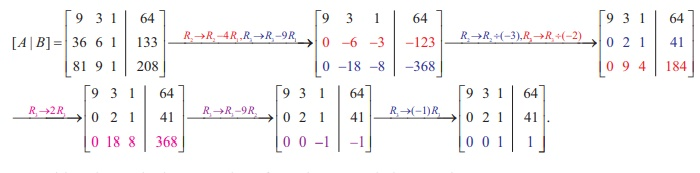Home | | Maths 12th Std | Matrix: Gaussian Elimination Method

# Matrix: Gaussian Elimination Method

This method can be applied even if the coefficient matrix is singular matrix and rectangular matrix.

Gaussian Elimination Method

This method can be applied even if the coefficient matrix is singular matrix and rectangular matrix. It is essentially the method of substitution which we have already seen. In this method, we transform the augmented matrix of the system of linear equations into row-echelon form and then by back-substitution, we get the solution.

### Example 1.27

Solve the following system of linear equations, by Gaussian elimination method :

4x + 3y + 6z = 25, x + 5 y + 7z = 13, 2x + 9 y + z = 1.

### Solution

Transforming the augmented matrix to echelon form, we getThe equivalent system is written by using the echelon form:

x + 5y  + 7z = 13 , ŌĆ” (1)

17y + 22z = 27 , ŌĆ” (2)

199z = 398 . ŌĆ” (3)Substituting z = 2, y = -1  in (1), we get x = 13 - 5  ├Ś (ŌłÆ1 ) ŌłÆ 7 ├Ś 2 = 4 .

So, the solution is ( x =4, y = - 1, z = 2 ).

Note. The above method of going from the last equation to the first equation is called the method of back substitution.

### Example 1.28

The  upward  speed  v(t) of a rocket  at time t is approximated by v(t) = at2 + bt + c, 0 Ōēż  t Ōēż 100 where a, b, and c are constants. It has been found that the speed at times t = 3, t = 6 , and t = 9 seconds are respectively, 64, 133, and 208 miles per second respectively. Find the speed at time  t = 15 seconds. (Use Gaussian elimination method.)### Solution

Since v(3) =64, v(6) = 133 and v(9) = 208 , we get the following system of linear equations

9a +3b + c = 64 ,

36a + 6b + c = 133,

81a + 9b + c = 208 .

We solve the above system of linear equations by Gaussian elimination method.

Reducing the augmented matrix to an equivalent row-echelon form by using elementary row  operations, we getWriting the equivalent equations from the row-echelon matrix, we get

9a + 3b + c =  64, 2b + c = 41, c= 1.

By back substitution, we getSo, we get v (t) = 1/3 t2  + 20t + 1.

Hence, v(15) = 1/3 (225) + 20(15) + 1 = 75 + 300 + 1 = 376

Tags : Definition, Solved Example Problems , 12th Mathematics : UNIT 1 : Applications of Matrices and Determinants
Study Material, Lecturing Notes, Assignment, Reference, Wiki description explanation, brief detail
12th Mathematics : UNIT 1 : Applications of Matrices and Determinants : Matrix: Gaussian Elimination Method | Definition, Solved Example Problems